AJP Excel Information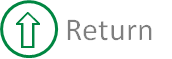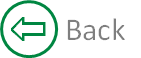### Custom leader lines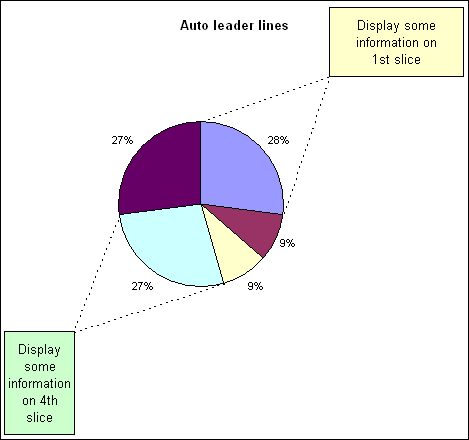##### Below is all the data and formula required to create the chart. The highlighted cells contain the actual charted data.
 A B C D E F 1 Auto leader lines % Angle Radius 2 a 3 0.272727273 98.18182 0.61 3 b 1 0.090909091 32.72727 4 c 1 0.090909091 32.72727 5 d 3 0.272727273 98.18182 6 e 3 0.272727273 98.18182 7 8 9 Slice X Y 10 1 90.00 3.74E-17 0.61 11 0.95 0.95 12 -8.18 0.603791 -0.086812051 13 14 4 -73.64 0.171857 -0.585290714 15 -0.95 -0.95 16 -171.82 -0.60379 -0.086812051
##### Formulas
 A B C D E F 1 Auto leader lines % Angle Radius 2 a 3 =B2/SUM(\$B\$2:\$B\$6) =360*D2 0.61 3 b 1 =B3/SUM(\$B\$2:\$B\$6) =360*D3 4 c 1 =B4/SUM(\$B\$2:\$B\$6) =360*D4 5 d 3 =B5/SUM(\$B\$2:\$B\$6) =360*D5 6 e 3 =B6/SUM(\$B\$2:\$B\$6) =360*D6 7 8 9 Slice X Y 10 1 90 =COS(RADIANS(B10))*\$F\$2 =SIN(RADIANS(B10))*\$F\$2 11 0.95 0.95 12 =B10-E2 =COS(RADIANS(B12))*\$F\$2 =SIN(RADIANS(B12))*\$F\$2 13 14 4 =90-SUM(E2:E4) =COS(RADIANS(B14))*\$F\$2 =SIN(RADIANS(B14))*\$F\$2 15 -0.95 -0.95 16 =B14-E5 =COS(RADIANS(B16))*\$F\$2 =SIN(RADIANS(B16))*\$F\$2

##### Select the range C10:D16 and use the chart wizard to create a standard xy-scatter chart.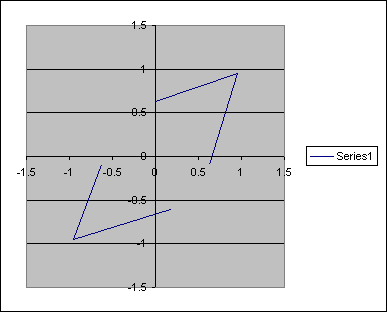##### Use the source data dialog to add another series to the chart.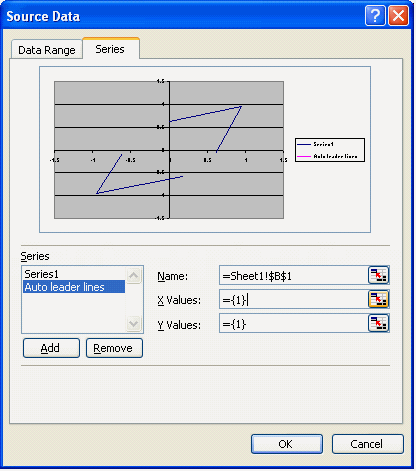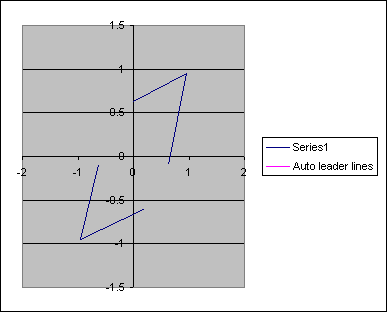##### Select the 2nd data series and change the chart type to pie.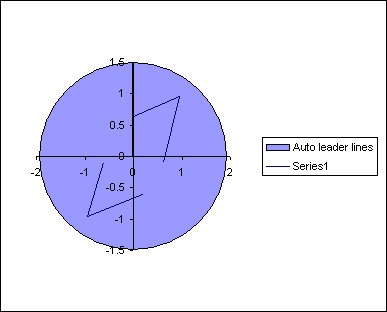##### Use the source data to specify the location of data, B2:B6, and labels, A2:A6, for the pie chart.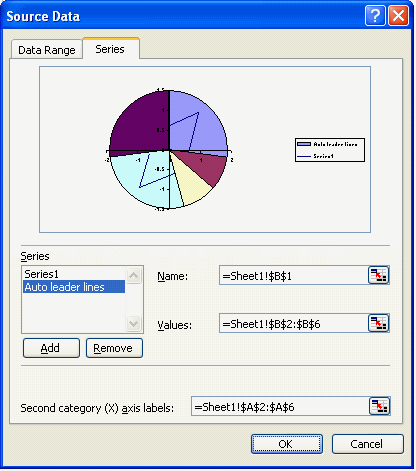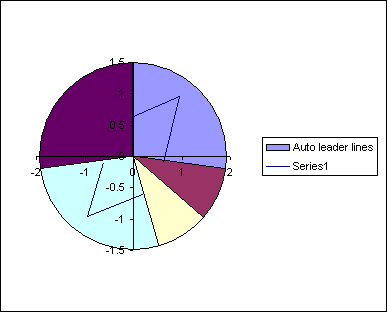##### Change the axis properties for both x and y axis. Set the minimum and maximum value to -1 and 1 respectively.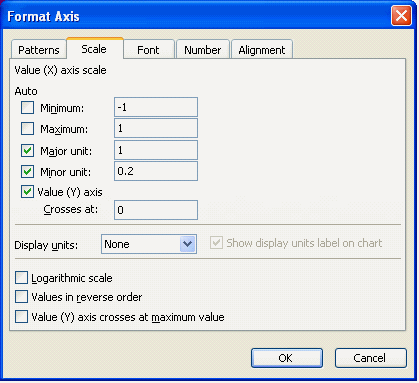##### The only problem with the chart in its current configuration is that the area available for the leader lines is restricted to the plot area, which is too close to the edge of the pie chart.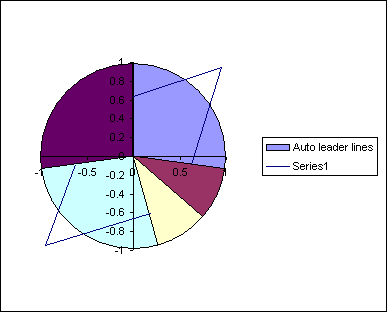##### To increase this area we need to explode and re assemble the pie chart. Select the pie and drag the slices away from the center. The further away you drag the slices the smaller the pie chart will end up.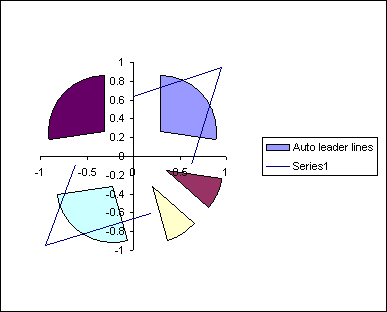##### After exploding and thereby reducing the size of the pie we need to drag the slice back together. If you do this whilst all the slices are selected then the pie will return to its original size. You have to do each piece individually. So select the pie and then select an individual slice. Drag this back to the center and then repeat for all the other pieces.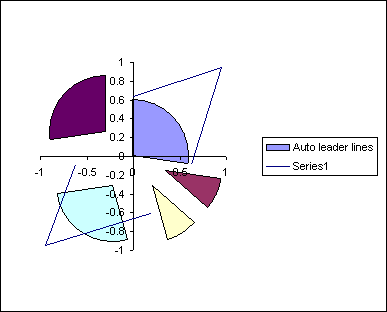##### The actual radius of the chart can be entered in to the cell F2 The end point of the leader lines, where the 2 lines meet, is controlled by the values used in cells C11:D11 and C15:D15. The position of contact of the leader lines with the pies circumference is calculated via the formulas.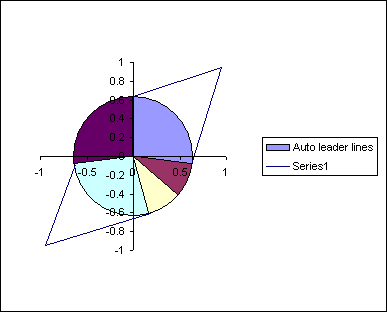##### Format both axis to remove lines, labels and tickmarks.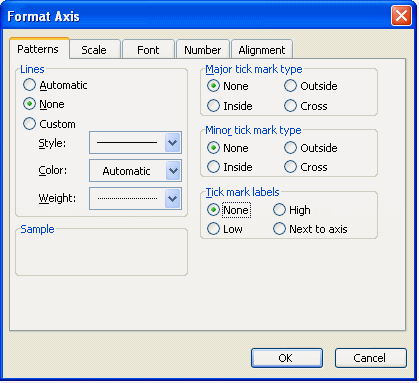##### You should now have a pie chart with leader lines for slices 1 and 4. This will automatically adjust for any changes in the pie charts data.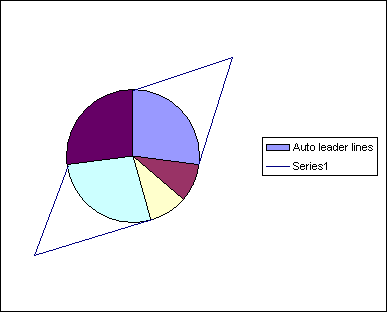##### Further formatting of the pie, leader lines, legend and chart can be applied. As can the textboxes used to hold any information needed.

Created August 2004
Last updated 5th August 2014

Microsoft® and Microsoft® Excel are registered trademarks of the Microsoft Corporation.
andypope.info is not associated with Microsoft. Copyright ©2007-2016 Andy Pope